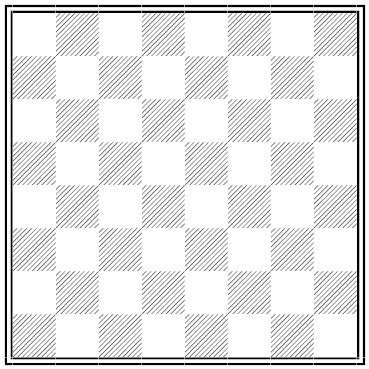# Even UpSuppose we cover a chessboard with 32 dominoes so that each domino covers two squares. What is the likelihood that there will be an even number of dominoes in each of the two orientations (horizontal and vertical)?

In fact this will always be the case. Consider the 32 squares in the odd-numbered horizontal rows. Each horizontal domino on the board covers either two of these squares or none of them. And each vertical domino covers exactly one of these squares. So the horizontal dominoes cover an even number of these squares (call it n), and the number of squares remaining in this group (32 – n) must also be even. This latter number is also equal to the number of vertical dominoes, so both quantities are even.

(By Vyacheslav Proizvolov.)